# How to define unit vector(â ) in LaTeX?

To define a unit vector with the help of latex, you need to be familiar with these three symbols given below.

 Vector \vec Hat \hat absalute value \left | ...\right |

Because the unit vector is identified by the above three symbols.

\documentclass{article}
\begin{document}
$\hat{a}=\frac{\vec{a}}{\left | \vec{a} \right |}$
\end{document}

Output :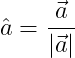## Rectangular unit vector components in LaTeX

The common three Rectangular unit vectors are marked with a cap symbol above the i, j and k symbol.

\documentclass{article}
\begin{document}
$\left ( \hat{i},\hat{j},\hat{k} \right )$
\end{document}

Output :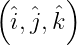In 3D space, the vector is always written as Rectangular unit vector.

\documentclass{article}
\begin{document}
$\vec{r}=x\hat{i}+y\hat{j}+z\hat{k}$
\end{document}

Output :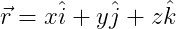For example, when defining a unit vector of a vector, it will be shaped

\documentclass{article}
\begin{document}
$\hat{r}=\frac{x\hat{i}+y\hat{j}+z\hat{j}}{\sqrt{x^{2}+y^{2}+z^{2}}}$
\end{document}

Output :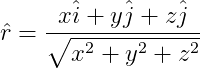In many high level physics books you will see that ex, ey and ez are used for rectangular unit vectors without using i, j and k.

\documentclass{article}
\begin{document}
$\left ( \hat{i},\hat{j},\hat{k} \right )$
\end{document}

Output :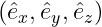All the commands used so far have been used in the program by default. And no external packages were installed.

You can install physics packages to define this unit vector in latex. Which contain \vu*{} command for unit vector symbol.

\documentclass{article}
\usepackage{physics}
\begin{document}
$\vu*{a}=\frac{\va*{a}}{\abs{\va*{a}}}$
\end{document}

Output :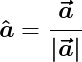Notice the latex program above. \va*{} and \abs{} commands are used for vector and absolute value symbols.#### Md Jidan Mondal

LaTeX expert with over 10 years of experience in document preparation and typesetting. Specializes in creating professional documents, reports, and presentations using LaTeX.